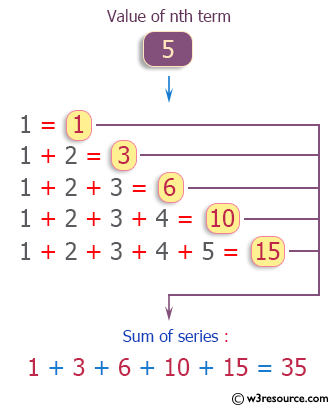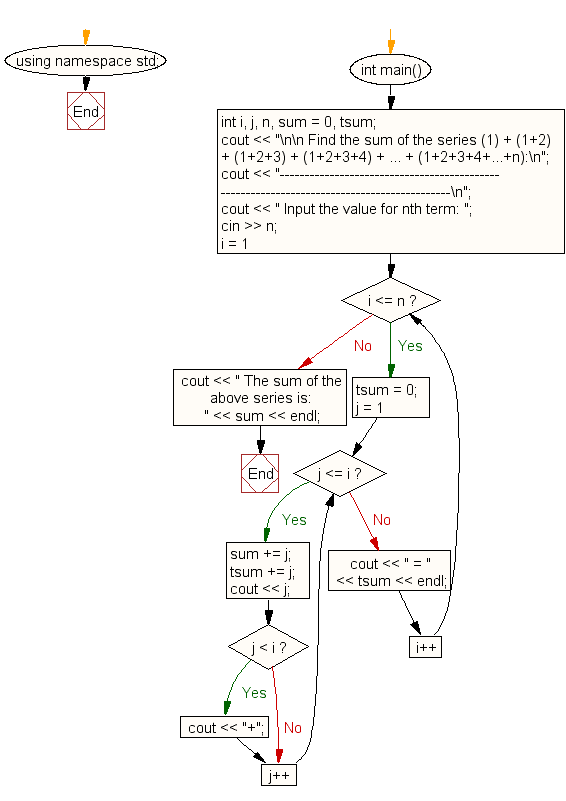﻿ C++ : Calculate the series (1) + (1+2) + … + (1+2+3+...+n)# C++ Exercises: Calculate the series (1) + (1+2) + (1+2+3) + (1+2+3+4) + ... + (1+2+3+4+...+n)

## C++ For Loop: Exercise-13 with Solution

Write a program in C++ to calculate the series (1) + (1+2) + (1+2+3) + (1+2+3+4) + ... + (1+2+3+4+...+n).

Pictorial Presentation:Sample Solution :-

C++ Code :

``````#include <iostream>
using namespace std;

int main()
{
int i, j, n, sum = 0, tsum;
cout << "\n\n Find the sum of the series (1) + (1+2) + (1+2+3) + (1+2+3+4) + ... + (1+2+3+4+...+n):\n";
cout << "------------------------------------------------------------------------------------------\n";
cout << " Input the value for nth term: ";
cin >> n;
for (i = 1; i <= n; i++)
{
tsum = 0;
for (j = 1; j <= i; j++)
{
sum += j;
tsum += j;
cout << j;
if (j < i)
{
cout << "+";
}
}
cout << " = " << tsum << endl;
}
cout << " The sum of the above series is: " << sum << endl;
}
``````

Sample Output:

``` Find the sum of the series (1) + (1+2) + (1+2+3) + (1+2+3+4) + ... + (
1+2+3+4+...+n):
-----------------------------------------------------------------------
-------------------
Input the value for nth term: 5
1 = 1
1+2 = 3
1+2+3 = 6
1+2+3+4 = 10
1+2+3+4+5 = 15
The sum of the above series is: 35
```

Flowchart:C++ Code Editor:

Contribute your code and comments through Disqus.

What is the difficulty level of this exercise?

﻿

## C++ Programming: Tips of the Day

What is a smart pointer and when should I use one?

This answer is rather old, and so describes what was 'good' at the time, which was smart pointers provided by the Boost library. Since C++11, the standard library has provided sufficient smart pointers types, and so you should favour the use of std::unique_ptr, std::shared_ptr and std::weak_ptr.

There was also std::auto_ptr. It was very much like a scoped pointer, except that it also had the "special" dangerous ability to be copied - which also unexpectedly transfers ownership.

It was deprecated in C++11 and removed in C++17, so you shouldn't use it.

```std::auto_ptr<MyObject> p1 (new MyObject());
std::auto_ptr<MyObject> p2 = p1; // Copy and transfer ownership.
// p1 gets set to empty!
p2->DoSomething(); // Works.
p1->DoSomething(); // Oh oh. Hopefully raises some NULL pointer exception.
```

Ref : https://bit.ly/3mc9GHE